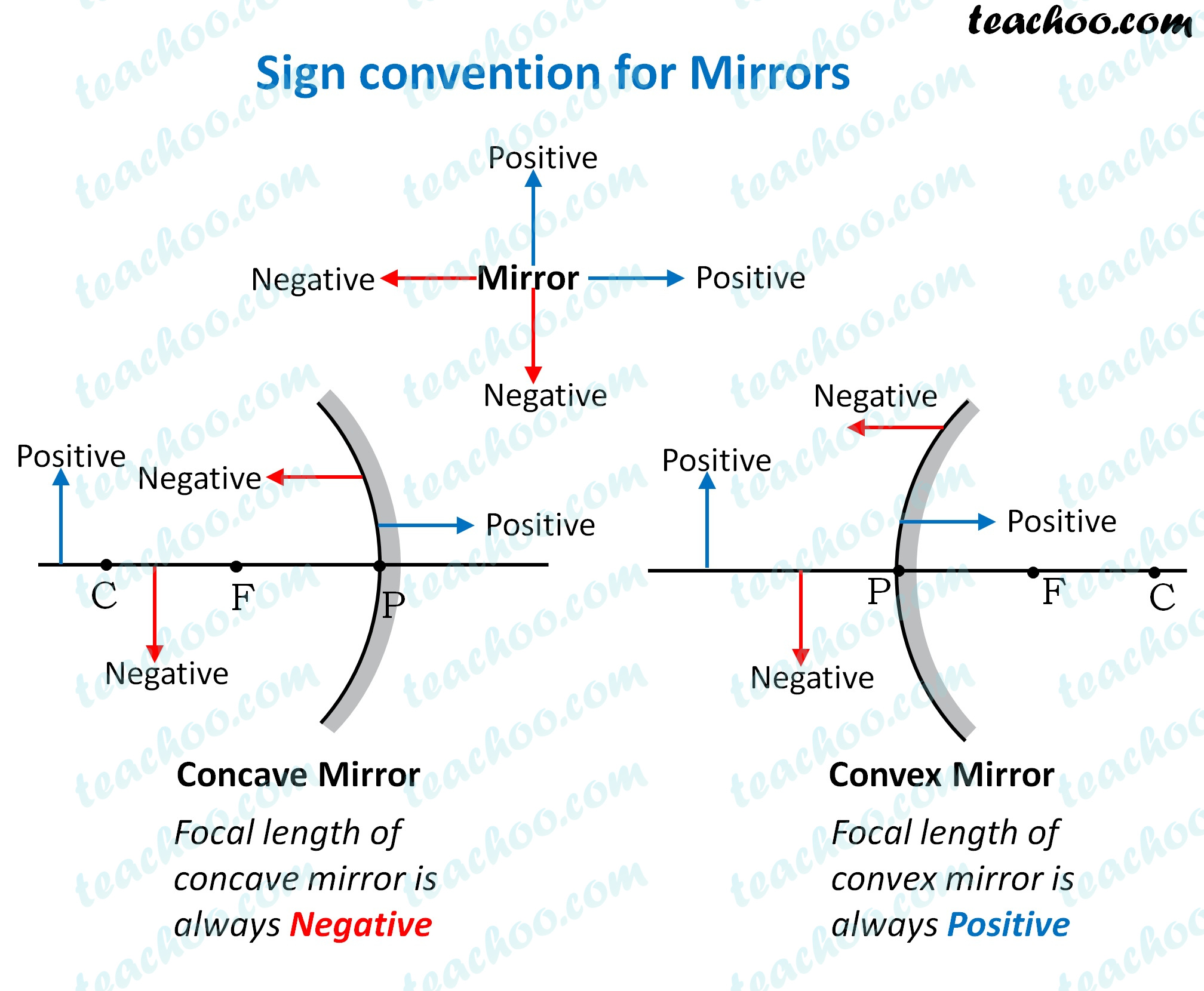Concepts

Class 10
Chapter 10 Class 10 - Light - Reflection and Refraction

In numericals, we need to calculate some things like

• Object distance
• Image distance
• Object height
• Image height
• Focal length

To calculate them correctly, we need to find whether it is positive or negative

The sign convention mentioned below helps us in itWe can see the sign convention in both mirrors

## Some points to note

• Since focus of concave mirror is on the left side (in front of the mirror) , Focal length of concave mirror is negative
• Since focus of convex mirror is on the right side (behind the mirror), Focal length of convex mirror is positive
• Since object is always in front of the mirror, object distance is always negative
• If a real image is formed, image is formed in front of the mirror... so image distance is negative
• If a virtual image is formed, image is formed behind the mirror... so image distance is positive
• Since object is always above the principal axis, object height will be positive
• If image is above the principal axis, image height will be positive. It means that image is erect
• If image is below the principal axis, image height will be negative. It means that image is inverted

Learn in your speed, with individual attention - Teachoo Maths 1-on-1 Class